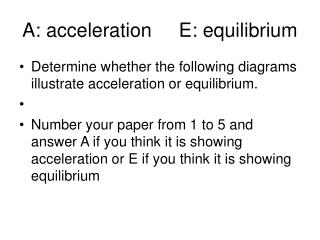DownloadDownload PresentationA: acceleration E: equilibrium

# A: acceleration E: equilibrium

Download Presentation## A: acceleration E: equilibrium

- - - - - - - - - - - - - - - - - - - - - - - - - - - E N D - - - - - - - - - - - - - - - - - - - - - - - - - - -
##### Presentation Transcript

1. A: acceleration E: equilibrium • Determine whether the following diagrams illustrate acceleration or equilibrium. • Number your paper from 1 to 5 and answer A if you think it is showing acceleration or E if you think it is showing equilibrium

2. A: acceleration E: equilibrium #1 10 N 10 N

3. #2 A: acceleration E: equilibrium 20 N 10 N

4. #3 A: acceleration E: equilibrium 50 N

5. #4 A: acceleration E: equilibrium 30 N 50 N 10 N

6. #5 A: acceleration E: equilibrium 40 N 10 N

7. #6 A: acceleration B: equilibrium • 1 20 N 10 N 10 N 20 N